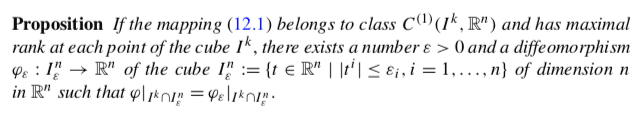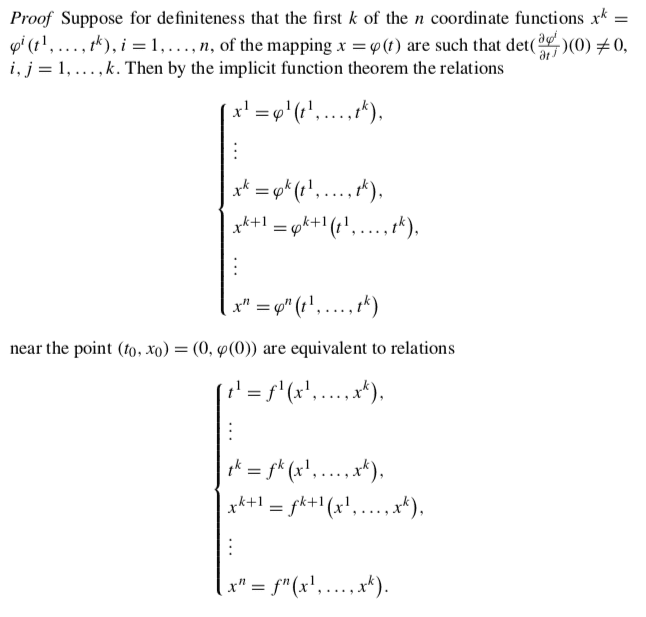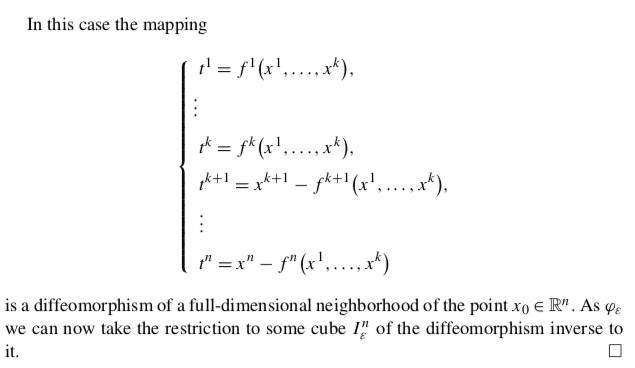# A Zorich proposition about local charts of smooth surfaces

Unconscious
From Zorich, Mathematical Analysis II, 1st ed., pag.163:where the referred mapping (12.1) is a map ##\varphi:I_k\to U_S(x_0)##, in which:

1. ##I_k\subset\mathbb{R}^n## is the k-dimensional unit cube,
2. ##x_0## is a generic point on the surface ##S## and ##U_S(x_0)## is a neighborhood of ##x_0## of points of ##S##.

I think that there is a deep significance in this theorem that I can't see yet, but anyway, looking brutally at the proof:we can see that up to this point the author uses the inverse function theorem to say that, if we consider only the first k coordinates both in the domain and in the range, ## \varphi ## is actually a diffeomorphism between two suitable neighborhoods ## U_{ \mathbb {R}^k_t} (0) ## e ## U _ {\mathbb {R} ^ k_x} (\varphi (0)) ##.
Then, the proof continues in this way:and here I don't understand why the whole ## f: U _ {\mathbb {R} ^ n_x} (\varphi (0)) \to U_ {\mathbb {R} ^ n_t} (0) ## (##n## instead of ##k##) should be a diffeomorphism. Indeed, it seems to me that ## t ^ {k + 1}, ..., t ^ n ## are always zero, as ##x## varies.

Staff Emeritus
Gold Member
Is your claim that ##f^l(x_1,...,x_n)=x^l## for ##l >k##? That seems unlikely (or you would have had something that was diffeomorphic on the whole space to begin with).

EDIT: sorry, I was too hasty. ##f^l## is only a function of ##x_1,...,x_k##, so could not possibly be equal to ##x_l## for ##l>k##.

To address your other possible confusion (though maybe you get the point and you're just not sure why it matters), the point of the theorem is if you have a diffeomorphism mapping to some lower dimensional manifold of ##\mathbb{R}^n## you can extend it (at least locally) to a diffeomorphism mapping to all of ##\mathbb{R}^n##.

It might help to consider an example. ##\phi(x,y)=(x,y,\sqrt{1-x^2-y^2})## is a function from a small square around the origin in ##\mathbb{R}^2## to a 2 dimensional manifold in ##\mathbb{R}^3##. All the proof is doing is saying you can turn this into ##\phi(x,y,z)=(x,y,z+\sqrt{1-x^2-y2})## and now it's a map from a small region around the origin in ##\mathbb{R}^3## to a 3 d submanifold of ##\mathbb{R}^3##.

Last edited:
Unconscious
Thank you, clear.